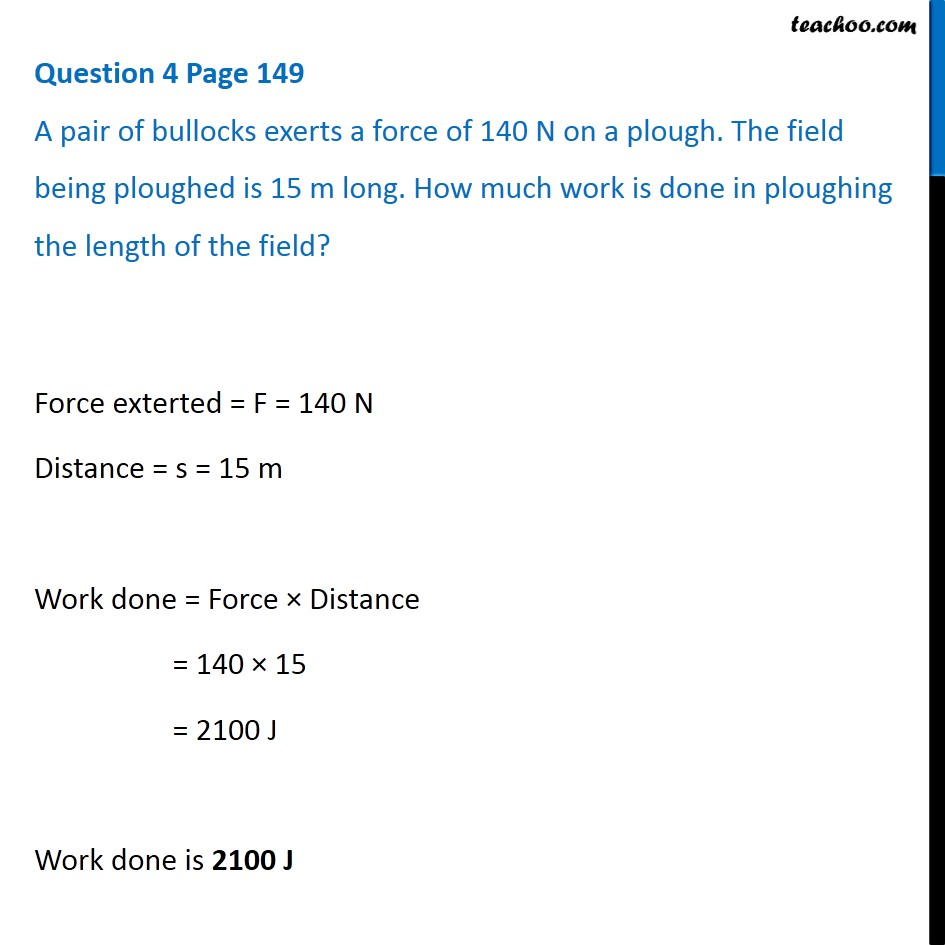Questions from Inside the chapter

Class 9
Chapter 11 Class 9 - Work and EnergyLearn in your speed, with individual attention - Teachoo Maths 1-on-1 Class

### Transcript

Question 4 Page 149 A pair of bullocks exerts a force of 140 N on a plough. The field being ploughed is 15 m long. How much work is done in ploughing the length of the field? Force exterted = F = 140 N Distance = s = 15 m Work done = Force × Distance = 140 × 15 = 2100 J Work done is 2100 J